## Landscape

Commands to load and display a real landscape

``````## Set briskaR working intern projection to LAMBERT 93 (default)
briskaRSetInternProjection(LAMBERT_93)

## load the maize_65 data set incorporated in briskaR
## in which polygons are either maize or non-maize fields
data(maize_65)

## then, randomly assign the GMO and non-GMO marks to maize fields
## non-maize fields being considered as receptors
initial_types=maize_65@thelandscape@data
nb_maize=sum(initial_types[,1])
nb_non_maize=sum(initial_types[,2])
types=as.data.frame(cbind(0,NA,initial_types[,2]))
names(types)=c("sources","neutral","receptors")
GM_fields=sample((1:nrow(types))[initial_types[,1]==1],
0.4*(nb_maize+nb_non_maize),replace=FALSE)
types[GM_fields,1]=1
types[,2]=1*(types[,1]==0 & types[,3]==0)

## generate land1 with the new marks for polygons
land1=maize_65
land1@thelandscape@data=types

## transform receptors at the border of the study domain
## into neutral cells
border_size=200
for(i in 1:land1@n){
if(land1@thelandscape@data[i,3]==1){
x0=land1@thelandscape@polygons[[i]]@Polygons[]@coords
if(sum(x0[,1]<land1@xmin+border_size | x0[,1]>land1@xmax-border_size |
x0[,2]<land1@ymin+border_size | x0[,2]>land1@ymax-border_size)>0){
land1@thelandscape@data[i,3]=0
land1@thelandscape@data[i,2]=1
}
}
}
## display the landscape
plot(land1)
``````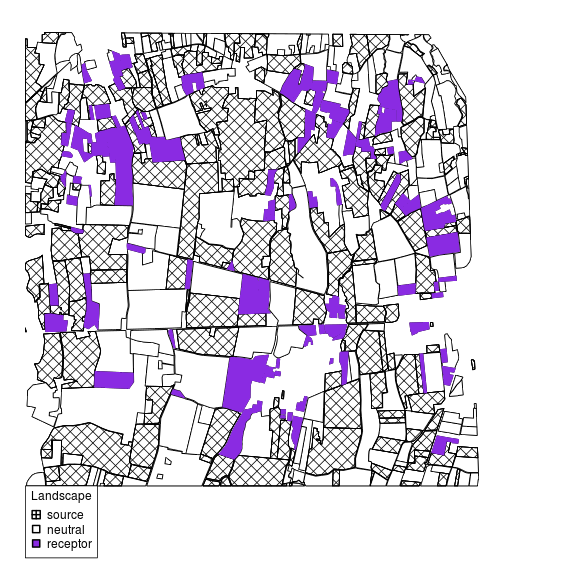Commands to generate and display a simulated landscape:

``````## generate the Voronoi tesselation
land=simulateInitialPartition(n=200, prop=0.4, range=10,
xmin=0, xmax=5000, ymin=0, ymax=5000)
## generate receptors at the borders of Voronoi cells
land2=simulateThickMargins(land, border_size=200, prob=0.5,
mean_thickness=5, v_thickness=50)
## display the landscape
plot(land2)
``````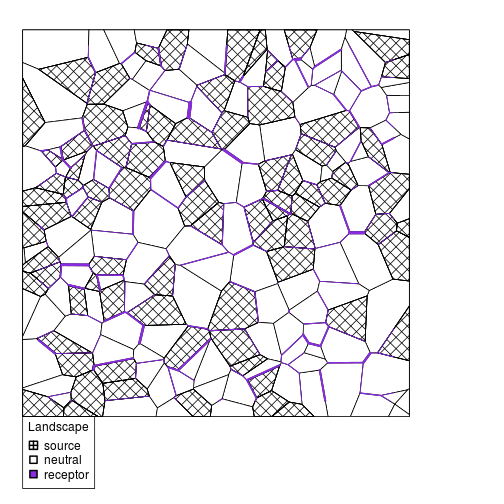## Individuals

Commands to generate and display exposed individuals for the real landscape `land1`:

``````## tuning parameters and variables
nb_ind=100 ; time_min=1 ; time_max=61
birth_dates=sample(time_min:time_max, size=nb_ind, replace=TRUE)
life_expectancies=rep(20, nb_ind)
toxic_gap=rep(15, nb_ind)
## generate exposed individuals
ind=simulateIndividuals(land1, n=nb_ind, mintime=time_min,
maxtime=time_max, dob=birth_dates,
life_duration=life_expectancies, toxic_threshold=toxic_gap)
## plot individuals in the landscape
plot(land1,ind)
``````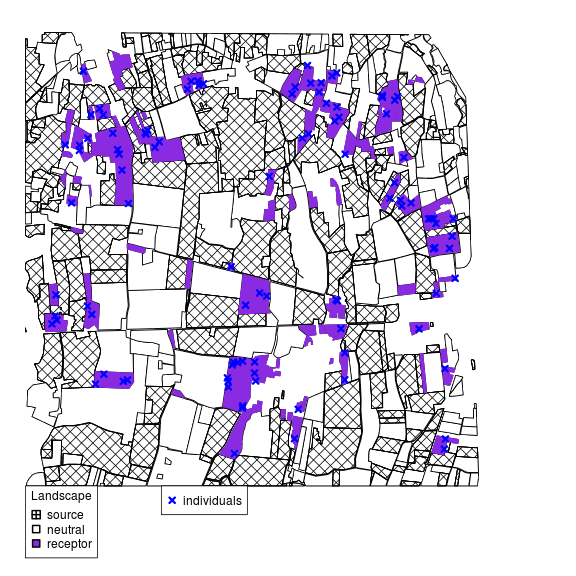## Toxic Intensity

Commands to compute the spatio–temporal quantities of GM pollen present on host plant leaves across the real landscape `land1` and the study period:

``````## generate emission functions for every sources
list_sources=getSPSources(land1)
emitted_pollen=create.pollen.sources(length(list_sources),time_max)
emitted_pollen=data.frame(t=emitted_pollen,
row.names=row.names(list_sources))
## load precipitation data and restrict them to June and July 2013
data(Precipitation)
precip=Precipitation\$RR[Precipitation\$AN==2013 & (Precipitation\$MOIS==6 | Precipitation\$MOIS==7)]
plot(1:61, precip, pch=19, xlab="Day", ylab="Rainfall intensity (in mm)")
``````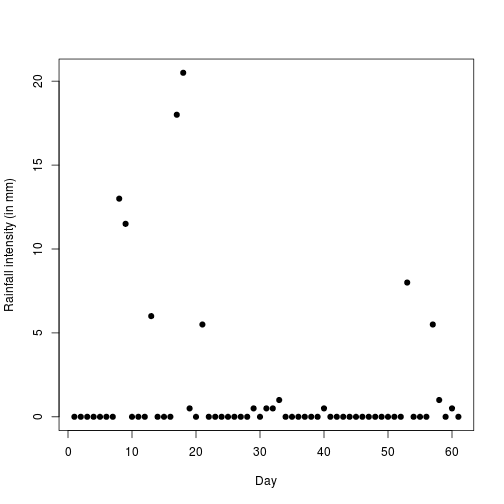``````## compute concentration of pollen across space and time
tox=toxicIntensity(objectL=land1, toxic_emission=emitted_pollen,
mintime=time_min, maxtime=time_max, size_raster=2^10,
alpha=list(minalpha=0.1, maxalpha=0.95, covariate_threshold=30,
simulate=FALSE, covariate=precip))
## display concentration of pollen at day 61
plot(x=land1, time=61, objectT=tox)
``````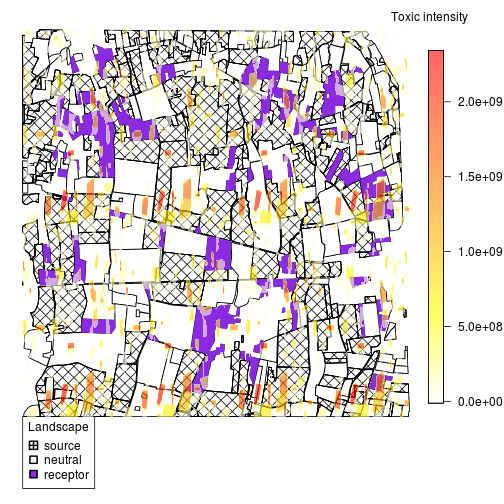## EcoToxicological

Commands to compute and display the internal concentration of Bt toxin and its impact for every host:

``````ind_impact=ecoToxic(land1, ind, tox, time_min, time_max,kin=4.19e-7, kout=0.1, deltat=0.1)
``````

Displaying the cumulative impact of the Bt toxin at time 61 can be done as follows:

``````#plot(x=land1, y=ind_impact, time=30, objectT=tox)
plot(x=land1, y=ind_impact, time=time_max, objectT=tox)
``````
``````plot(maize.landscape,maize.individuals,time=61,objectT=tox)
``````